# How long will students need to complete their work?

Education Perfect's Lists and Smart Lessons have an estimated completion time to help you with lesson planning.

When navigating through the Content Library, click on a list or lesson to see the estimated completion time. In the sidebar that appears, there will be a stopwatch icon with a number next to it.

## Calculating completion time

When a lesson is first created, the time is based on the number and type of slides within the lesson listed below. Once the first student has completed a new lesson, we use their completion time as an estimate. From there, we use the 80th percentile to estimate completion time.

 Information sections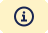Multiply the number of slides by 20. Vocabulary sections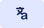Multiply the number of questions by 20. Skill Mastery sections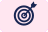Multiply the number of questions needed to complete the section by 40. Quiz sections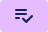Multiply the number of questions by 40. Extended Response sections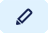Multiply the number of questions by 60.

## Peer Review

When assigning a Peer Review Task, you'll need to account for the additional time that this will take. The new estimated time will be shown in the Peer Review section while setting up the task.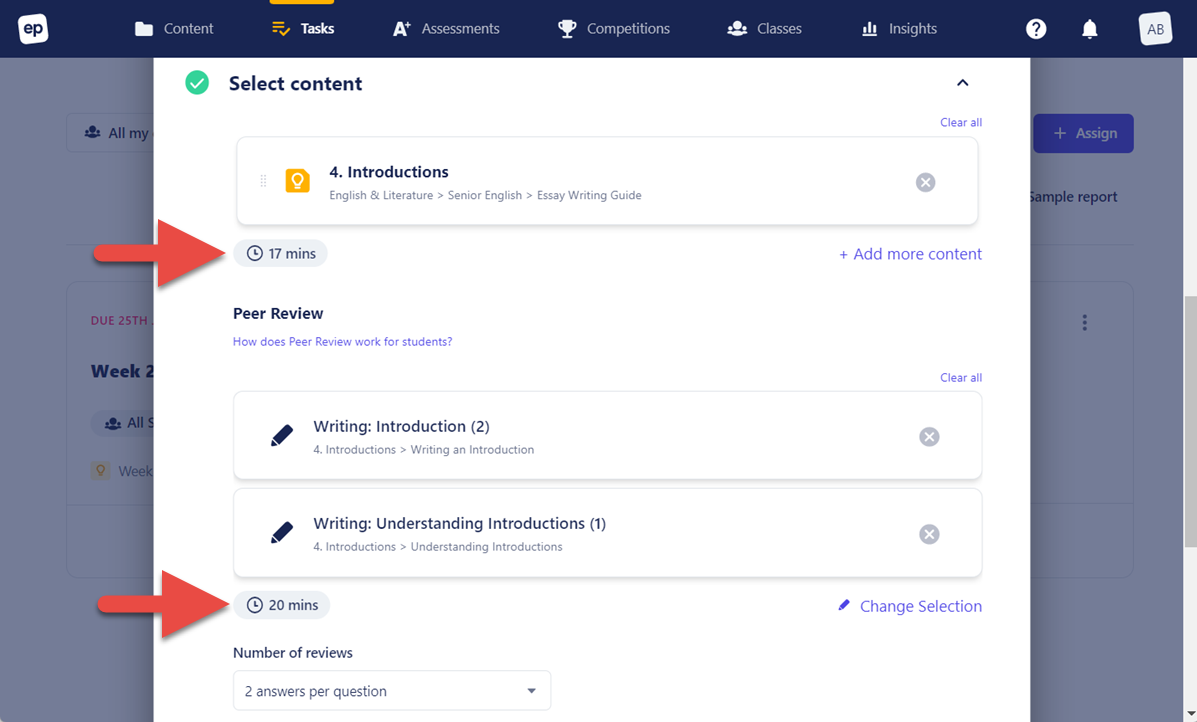2 minutes is added to the overall completion time for every Extended Response question students are required to leave star feedback on. 5 minutes is added for star  and comment feedback.